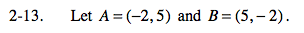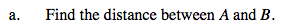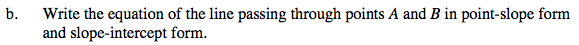### Home > PCT > Chapter Ch2 > Lesson 2.1.1 > Problem2-13

2-13.
1. Let A = (−2, 5) and B = (5, −2). Homework Help ✎

1. Find the distance between A and B.

2. Write the equation of the line passing through points A and B in point-slope form and slope-intercept form.Plot the points and connect them. Then create a right triangle with segment AB as the hypotenuse.
Use the Pythagorean Theorem to calculate the length of segment AB.1. Determine the slope: rise/run.
2. Substitute a given point and the slope into the point-slope formula.
3. Solve for y for the slope-intercept form.